# SeamusMcCabe

•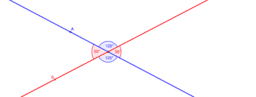### Theorem 01 - Vertically Opposite Angles

Activity

SeamusMcCabe

•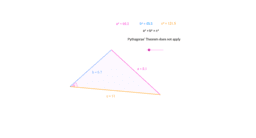### Theorem 15 - The Converse of Pythagoras' Theorem

Activity

SeamusMcCabe

•### Theorem 19 - Angles in a circle, standing on an arc.

Activity

SeamusMcCabe

•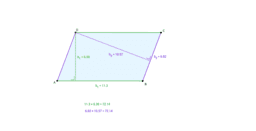### Theorem 18 - Area of a Parallelogram

Activity

SeamusMcCabe

•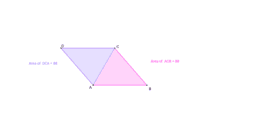### Theorem 17 - A Diagonal Bisects the Area of a Parallelogram

Activity

SeamusMcCabe

•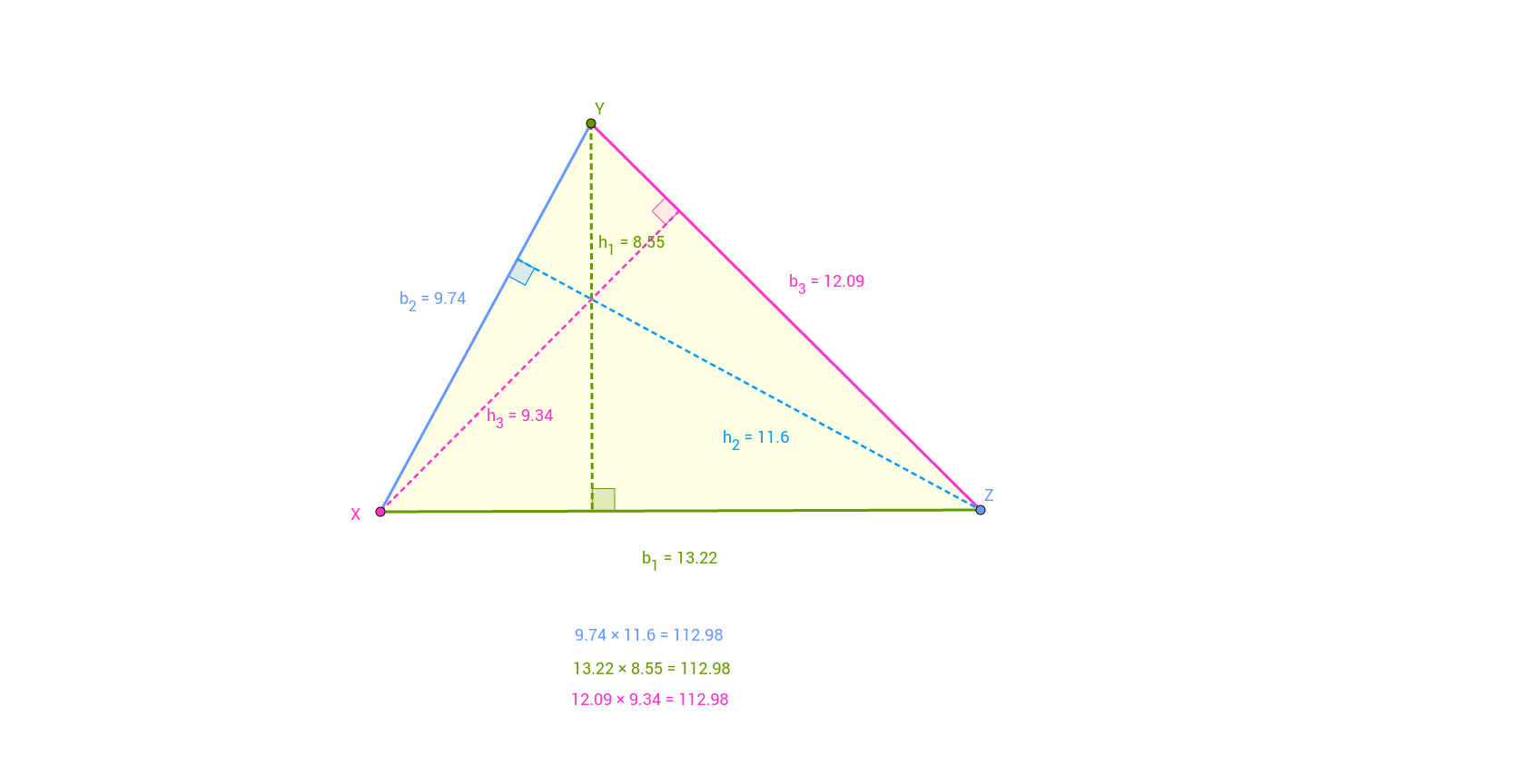### Theorem 16 - Base times Height in a Triangle

Activity

SeamusMcCabe

•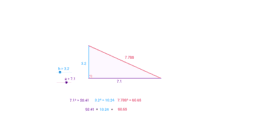### Theorem 14 - Pythagoras' Theorem

Activity

SeamusMcCabe

•### Theorem 13 - Similar Triangles

Activity

SeamusMcCabe

•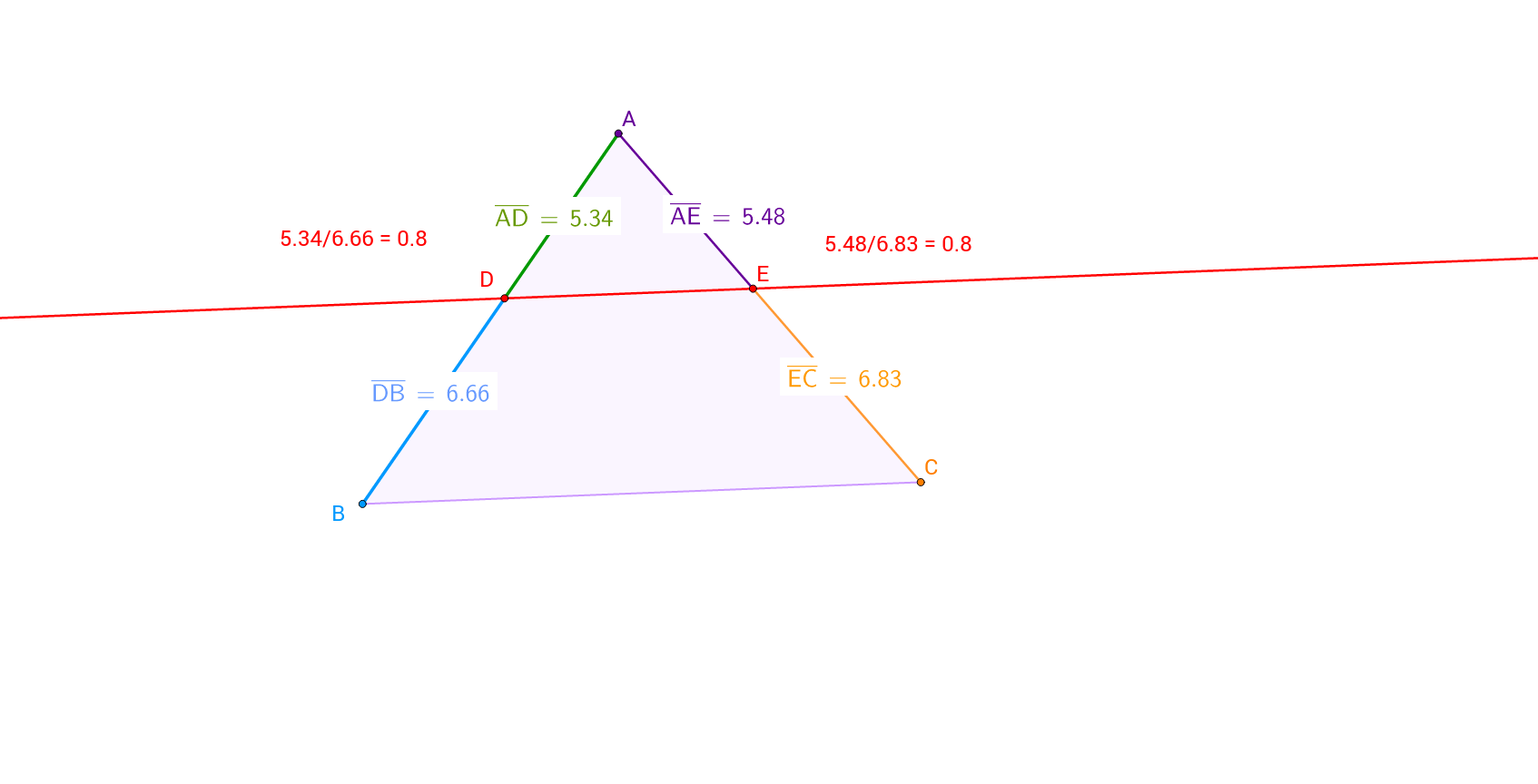### Theorem 12 - Two Sides of a Triangle cut by a line Parallel

Activity

SeamusMcCabe

•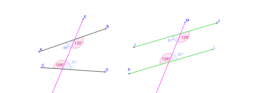### Theorem 03 - Alternate Angles and Parallel Lines

Activity

SeamusMcCabe

•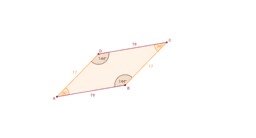### Theorem 09 - Opposite Sides and Angles in a Parallelogram

Activity

SeamusMcCabe

•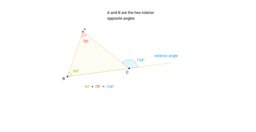### Theorem 06 - The Exterior Angle of a Triangle

Activity

SeamusMcCabe

•### Theorem 02 - Isosceles Triangle

Activity

SeamusMcCabe

•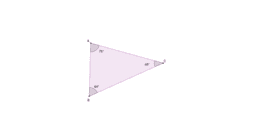### Theorem 04 - Angles in a Triangle

Activity

SeamusMcCabe

•### Theorem 07 - Comparing Sides and Angles in a Triangle

Activity

SeamusMcCabe

•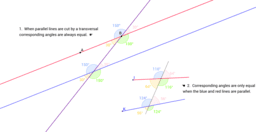### Theorem 05 - Corresponding Angles

Activity

SeamusMcCabe

•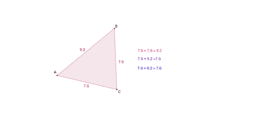### Theorem 08 - The Sum of Two Sides of a Triangle

Activity

SeamusMcCabe

•### Theorem 11 - Three Parallel Lines Cutting a Transversal

Activity

SeamusMcCabe

•Activity

SeamusMcCabe